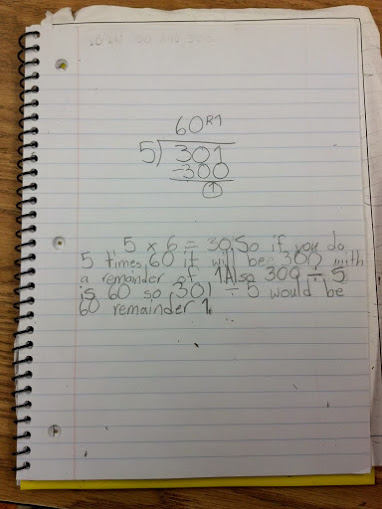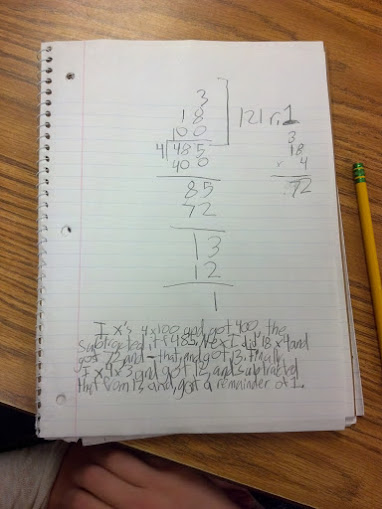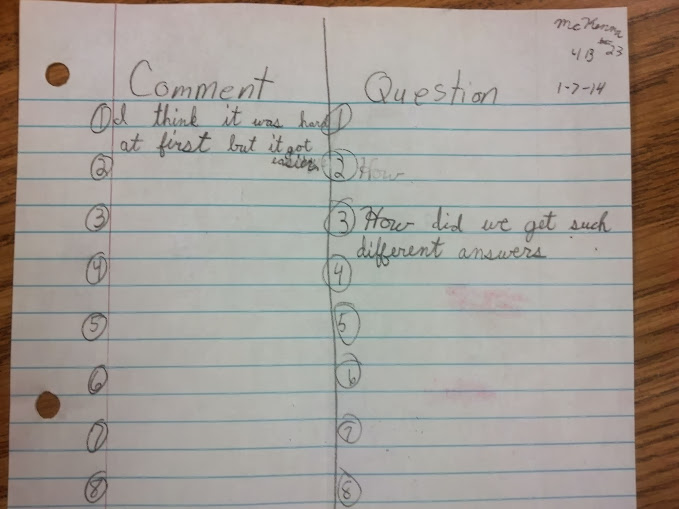# Problem Solving Division Day 1

7 teachers like this lesson
Print Lesson

## Objective

SWBAT solve word problems using division skills.

#### Big Idea

In this fun partner based lesson, students solve multistep word problems and practice discussing division and multiplication strategies.

## Number Hook

5 minutes

I start today's math lesson with this number hook. I tell students that I will be able to tell them their age and address simply by having them write down some numbers and perform a few simple calculations.

I tell the students these instructions:

1) Write down their street number: 2979
2) Double it: 5958
3) Add 5: 5963
4) Multiply by 50: 298150
5) Add the person’s age (31): 2981181
6) Add the number of days in a year (365): 298546

Students tell me their result.  I subtract 15 from the last two numbers and get the student's age.  Then I subtract 6 from the remaining other digits to get the person’s address.

For example, let's suppose someone lived at 1234 Apple Street who was 54. Their numbers would look like this from the above directions.

1. 1234

2. 2468

3. 2468 + 5 = 2473

4. 2473 * 50 = 123650  (note, my students did get nervous about multiplying four digits by two digits, but a few students were able to tell the rest of the class that that they could multiply the number by five and then multiply that number by 10)

5. 123650 + 54 = 123704

6. 123704 + 365 = 124069

Result: 69 - 15 = 54 (the person's age) and 1240 - 6 = 1334

My students love this trick. They think it's so "cool" that math can tell their age and address. They do understand that there is some sort of pattern happening to the numbers in order to get their age and address, but they don't really know what.  I do not think it is necessary they know the "how" just that math is everywhere - even in magic!

## Warm Up

5 minutes

For this warm up, I challenge students to write a division problem using a 3-digit dividend and one digit divisor that results in a quotient and a remainder of 1. I ask them to show all their work.

This is an example of a student's work. I think the written explanation is somewhat confusing, but you can see by the division problem that this student's work seems to have a solid understanding of multiples of ten with division and how to result with a remainder of 1.This is another student's thinking. This student admitted that this division problem was a lucky guess.  This student was amazed at the other strategies his/her peers discussed when talking about this problem because the student really thought that his was purely a guess and check type of problem. That told me a lot about this student's number sense right now and where this student is at in thinking about numbers.One of the most surprising aspects to this journal activity was the amount of thinking my students did.  One student reported that he knew that if the divisor was 2, the biggest remainder in that problem would be a one. He went on to say that all he needed was an odd number since all even numbers are divisible by 2.  I was really in awe of his thinking and so proud of the way he communicated it. (So sad I didn't have my video rolling to capture it)

Another student reported that she knew her multiples of ten and that she could simply add one more any multiple of ten to get a remainder of 1. She gave this example, "I know five times six equal thirty. So, fifty times six equals three hundred which means five hundred times 6 equals three thousand. I know I just need to make three thousand one and divide it by six to get a remainder of 1!"

## Concept Development

45 minutes

Partner work is a strategy that promotes participation and interaction that I try to incorporate on a regular basis.  It fosters a deeper and more active learning process, and it also provides me with valuable information about what students understand regarding topics or concepts. In addition to exposing students to different approaches and ways of thinking, working with another student can promote a sense of belonging. Working together with a partner also gives students the opportunity to learn from and teach each other.

For this lesson, students will use a strategy called Thinking- Aloud Partner Problem Solving. I wanted to incorporate partner work in this lesson, but also use problem solving situations in which students would have opportunities to apply the division skills they have gained from this division unit. Problem solving is an integral part of my students learning. Students  acquire ways of thinking, habits of persistence and curiosity, and confidence in unfamiliar situations that will serve them well outside the mathematics classroom. In everyday life and in the workplace, being a good problem solver can lead to great advantages.

The goal for today's lesson is for partners to work on the problems presented on the 3 ÷ 1 (With Remainder) problem set worksheet.

I know that students will not finish this worksheet in the time allotted, but I encourage them to work wisely and efficiently so they are able to continue to work during tomorrow's lesson.  As students work, I am constantly moving around the classroom to observe students thinking. As much as I can, I resist the urge to re-direct students thinking until I am certain they have talked with their learning partner about the problem. By doing this, students partners often help correct misunderstandings and errors.

Students utilize Math Practice Standards 1 and 3 in this lesson. CCSS MP1 states mathematically proficient students start by explaining to themselves the meaning of a problem and looking for entry points to its solution. They analyze givens, constraints, relationships, and goals. They make conjectures about the form and meaning of the solution and plan a solution pathway rather than simply jumping into a solution attempt. They consider analogous problems, and try special cases and simpler forms of the original problem in order to gain insight into its solution. They monitor and evaluate their progress and change course if necessary. Mathematically proficient students check their answers to problems using a different method, and they continually ask themselves, “Does this make sense?” They can understand the approaches of others to solving complex problems and identify correspondences between different approaches.

One of the strengths in this lesson is directly related to the above text. The think aloud partner problem solving (TAPPS) instructional strategy allows and encourages students to share their thinking with peers and allows peers to help or guide checking work and the reasonableness of answers.  Student partners can continually check each other's work and ask each other if their answers makes sense.  Often times, one student's strategy or method differs from their partners, which creates an opportunity to see and hear other approaches and build students' abilities to think flexibly.

Below, you can see two students using the TAPPS strategy and talking through a problem.

In this student's work, you can see that a question this student had was, "How did we get such different answers?"The above example is exactly what I wanted my students to be able to do in this lesson, thoughtfully reflect on his or her partners thinking in order to deepen understanding of division.

Students work until the end of the class period.